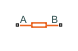# Thermal Resistor

Heat transfer by conduction through a layer of material

•Libraries:
Simscape / Electrical / Passive / Thermal

## Description

The Thermal Resistor block represents heat transfer by conduction through a layer of material. The heat transfer is:

• Governed by Fourier’s law

• Proportional to the temperature difference across the layer of material

• Inversely proportional to the thermal resistance of the material

The equation for conductive heat transfer is:

`${Q}_{AB}=\frac{{T}_{AB}}{{R}_{thermal}},$`

where:

• QAB is the heat flow through the material.

• TAB is the temperature difference across the layer of material.

• Rthermal is the thermal resistance of the material.

Thermal resistance can be calculated as:

`${R}_{thermal}=\frac{D}{kA},$`

where:

• D is the thickness of the layer of material.

• k is the thermal conductivity of the material.

• A is the area normal to the heat flow direction.

Use the Thermal Resistor block to parameterize an equivalent component in terms of thermal resistance of the material layer. To parameterize an equivalent component in terms of the thickness, thermal conductivity, and area of the material layer, use the Conductive Heat Transfer block from the Simscape™ Foundation library.

### Variables

To set the priority and initial target values for the block variables prior to simulation, use the Initial Targets section in the block dialog box or Property Inspector. For more information, see Set Priority and Initial Target for Block Variables.

Nominal values provide a way to specify the expected magnitude of a variable in a model. Using system scaling based on nominal values increases the simulation robustness. Nominal values can come from different sources, one of which is the Nominal Values section in the block dialog box or Property Inspector. For more information, see System Scaling by Nominal Values.

## Ports

### Conserving

expand all

Thermal conserving port associated with surface A of the material that the heat flows through.

Thermal conserving port associated with surface B of the material that the heat flows through.

## Parameters

expand all

Thermal resistance, Rthermal.

## Version History

Introduced in R2016a